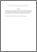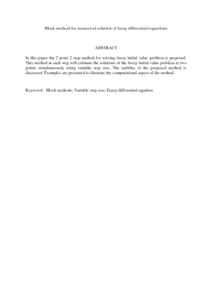# Block method for numerical solution of fuzzy differential equations

## Citation

Mehrkanoon, Siamak and Suleiman, Mohamed and Abdul Majid, Zanariah (2009) Block method for numerical solution of fuzzy differential equations. International Mathematical Forum, 4 (45-48). pp. 2269-2280. ISSN 1312-7594; ESSN: 1314-7536

## Abstract

In this paper the 2 point 2 step method for solving fuzzy initial value problem is proposed. This method at each step will estimate the solutions of the fuzzy initial value problem at two points simultaneously using variable step size. The stability of the proposed method is discussed. Examples are presented to illustrate the computational aspect of the method.Preview
PDF (Abstract)
Block method for numerical solution of fuzzy differential equations.pdfView Item